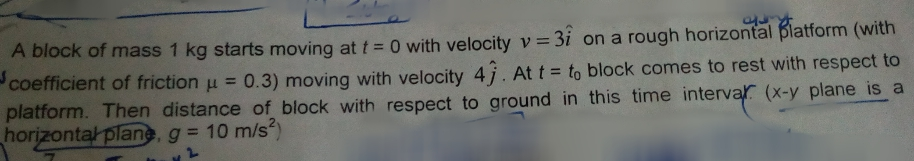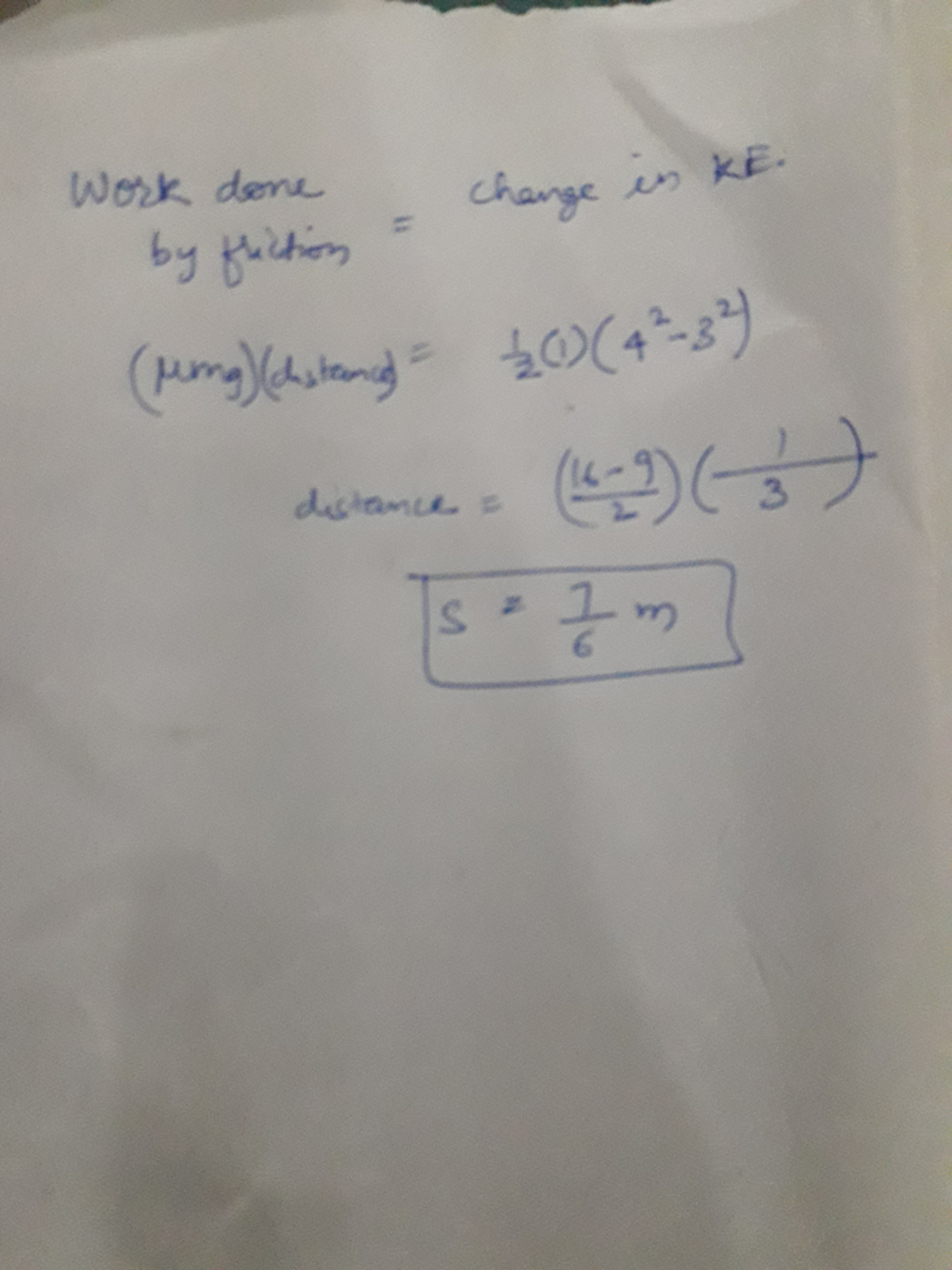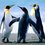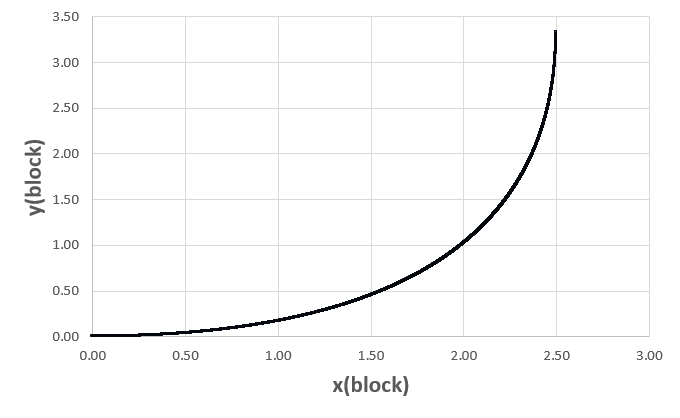# Classical mechanics problemNote by Kudo Shinichi
2 years, 1 month ago

This discussion board is a place to discuss our Daily Challenges and the math and science related to those challenges. Explanations are more than just a solution — they should explain the steps and thinking strategies that you used to obtain the solution. Comments should further the discussion of math and science.

When posting on Brilliant:

• Use the emojis to react to an explanation, whether you're congratulating a job well done , or just really confused .
• Ask specific questions about the challenge or the steps in somebody's explanation. Well-posed questions can add a lot to the discussion, but posting "I don't understand!" doesn't help anyone.
• Try to contribute something new to the discussion, whether it is an extension, generalization or other idea related to the challenge.
• Stay on topic — we're all here to learn more about math and science, not to hear about your favorite get-rich-quick scheme or current world events.

MarkdownAppears as
*italics* or _italics_ italics
**bold** or __bold__ bold
- bulleted- list
• bulleted
• list
1. numbered2. list
1. numbered
2. list
Note: you must add a full line of space before and after lists for them to show up correctly
paragraph 1paragraph 2

paragraph 1

paragraph 2

[example link](https://brilliant.org)example link
> This is a quote
This is a quote
    # I indented these lines
# 4 spaces, and now they show
# up as a code block.

print "hello world"
# I indented these lines
# 4 spaces, and now they show
# up as a code block.

print "hello world"
MathAppears as
Remember to wrap math in $$ ... $$ or $ ... $ to ensure proper formatting.
2 \times 3 $2 \times 3$
2^{34} $2^{34}$
a_{i-1} $a_{i-1}$
\frac{2}{3} $\frac{2}{3}$
\sqrt{2} $\sqrt{2}$
\sum_{i=1}^3 $\sum_{i=1}^3$
\sin \theta $\sin \theta$
\boxed{123} $\boxed{123}$

## Comments

Sort by:

Top Newest

$\vec v_b=3 \vec{i}, \vec{a}= \dfrac{3(-3 \vec i +4 \vec j)}{5}\, |\vec a|=3, |\vec v_{b/p}|=5 \implies t_0=5/3 s.$ (Using formula $0-5=-3t_0$) For block, $\vec{s}_b=3t_0 \vec i + (1/2) \vec{a} {t_0}^2$ Use this to get $\vec{s}=5 \vec i + \dfrac{10}{3} \vec j$ Note: $v_b$ is velocity of block in ground frame, $v_{b/p}$ is velocity of block in platform frame.

- 1 year, 7 months ago

Log in to reply

Here is my solution

Initial assumptions:

1) The initial speed of the block is $(3,0)$ with respect to the ground
2) The initial position of the block is $(0,0)$ with respect to the ground
2) The speed of the platform is $(0,4)$ with respect to the ground
3) The friction force has a magnitude equal to the $\mu \, m \, g$, and a direction opposite to the relative velocity of the block with respect to the platform. Both of these velocities are given with reference to ground. In other words:

$\vec{F}_{friction} \propto \, -(\vec{v}_{block} - \vec{v}_{platform})$

Running the simulation over time results in the block coming to rest relative to the platform after $1.666$ seconds, at which time the $x$ coordinate of the block is $2.5$ with respect to ground, and the $y$ coordinate is $3.333$ with respect to ground. The block is a distance $4.1666$ from the origin, and it has moved a total arc length of $4.753$ with respect to ground.

The $(x,y)$ trajectory of the block relative to ground is shown below:- 2 years, 1 month ago

Log in to reply

@Steven Chase sir please help with this problem discussion on a David Morin Question.

- 1 year, 6 months ago

Log in to reply

Sir initial speed of block should be wrt platform . As such in ground frame it has initial speed to be net 5

- 2 years, 1 month ago

Log in to reply

If that is the case, my last paragraph changes as follows:

Running the simulation over time results in the block coming to rest relative to the platform after $1$ second, at which time the $x$ coordinate of the block is $1.5$ with respect to ground, and the $y$ coordinate is $4$ with respect to ground. The block is a distance $4.272$ from the origin, and it has moved a total arc length of $4.348$ with respect to ground.

- 2 years, 1 month ago

Log in to reply

Sir but my energy method I am getting 7/6m which is actually the answer given sir.

- 2 years, 1 month ago

Log in to reply

Perhaps you can post your solution

- 2 years, 1 month ago

Log in to reply

This is by energy method sir . Sir I have posted above with the question.

- 2 years, 1 month ago

Log in to reply

@Steven Chase Sir I have posted my energy method above with the question.

- 2 years, 1 month ago

Log in to reply

@Steven Chase Sir can u pls tell.is there anything wrong I did ?

- 2 years, 1 month ago

Log in to reply

I wanted to check my original solution, since it was a bit involved. So then I remembered the first postulate of special relativity: "The laws of physics are the same in all inertial frames of reference". Since the platform is not accelerating, an observer sitting on the platform and watching should observe "ordinary" physics. And we can apply simple kinematics to that case. Let $v_0$ be the initial velocity of the block relative to the platform. There is a constant acceleration relative to the platform equal to $\mu \, g$.

$\frac{v_0}{\mu \, g} = t_f \\ t_f = 1$

Then the distance the block slides relative to the platform is:

$D = \frac{1}{2} \, \mu \, g \, t_f^2 = \frac{3}{2}$

And over the one second, the platform has moved 4 meters. So the final position of the block with respect to the ground is $(\frac{3}{2}, 4)$. This matches the more complicated approach I took earlier.

Then, to find the total arc length traveled with respect to ground, begin with the expressions for the velocity relative to ground.

$(v_x,v_y) = (3 - 3 t, 4) \\ v = \sqrt{25 - 18t + 9 t^2}$

The total arc length is:

$L = \int_0^1 \sqrt{25 - 18t + 9 t^2} \, dt = 4.3484$

This also matches the result of my previous solution

- 2 years, 1 month ago

Log in to reply

Can it be solved without energy method ? @Steven Chase sir @Aaghaz Mahajan bro ??

- 2 years, 1 month ago

Log in to reply

Which dimension is gravity in (i, j, or k)?

- 2 years, 1 month ago

Log in to reply

Sir, it is written that x-y plane is a horizontal plane, so I think g should be in k.....

- 2 years, 1 month ago

Log in to reply

Ok, thanks

- 2 years, 1 month ago

Log in to reply

@Steven Chase sir u got the answer? By other method?

- 2 years, 1 month ago

Log in to reply

I have posted my solution

- 2 years, 1 month ago

Log in to reply

Yes........I mean, I solved it using relative velocity.......View the motion of the block with respect to the horizontal platform........The time after which it stops is 1.66666 seconds.....I think you can proceed from here onwards....:)

- 2 years, 1 month ago

Log in to reply

@Aaghaz Mahajan bro but then from that frame answer I am getting is wrong , can u tell what r u getting ?? Its difficult for me to get answer using relative frame I used that. .

- 2 years, 1 month ago

Log in to reply

Well, what did you try??? What is your answer??

- 2 years, 1 month ago

Log in to reply

I am getting answer as 7/6 m from energy method , but not from this . Can u show by relative frame @Aaghaz Mahajan bro

- 2 years, 1 month ago

Log in to reply

Well, what is the correct answer??? I am getting the answer to be 5 m.........Here is my method:- Taking the velocity of block wrt to the platform, we get it to be <3,-4> i.e. v = 5 m/s. Also, the acceleration is -3 m/s^2. So, the time taken is 1.66666666 seconds. Now, the displacement of the block in that time is <5,-20/3> and the displacement of the platform is <0,4>. Using relative velocity again, we get the displacement of the block wrt ground to be <5,0>......

- 2 years, 1 month ago

Log in to reply

Answer is 7/6 m only @Aaghaz Mahajan bro. We need total distance not displacement

- 2 years, 1 month ago

Log in to reply

@Steven Chase Sir u r method is correct , but what's incorrect in my method (energy)??

- 2 years, 1 month ago

Log in to reply

I have several comments:

1) I interpret the "4" in your energy expression as being the final velocity with respect to ground, which is the same velocity as the platform. It's important to note that it is relative to ground.

2) The "3" in your energy expression is relative to the platform (per your previous clarification). So you have two different references in your energy equation, which seems problematic.

3) The other concern is that the work calculation has the following general form:

$W = \int \vec{F} \cdot \vec{d \ell}$

If the force magnitude is constant AND the force is everywhere parallel to the motion, this reduces to:

$W = |\vec{F}| \, \Delta \ell$

How do you know that these simplifications should occur?

- 2 years, 1 month ago

Log in to reply

Seems like 1 is causing trouble to my energy method , will try again

- 2 years, 1 month ago

Log in to reply

×

Problem Loading...

Note Loading...

Set Loading...